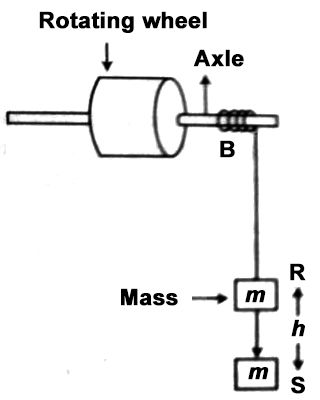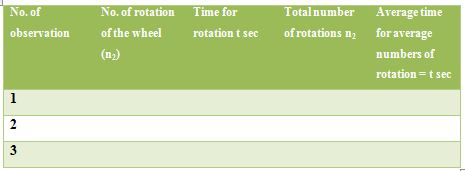Physics

# Experiment: Determination of Moment of Inertia of a Fly WheelExperiment: Determination of moment of Inertia of a Fly Wheel

Theory: The flywheel consists of a weighty round disc/massive wheel fixed with a strong axle projecting on either side. The axle is mounted on ball bearings on two fixed supports. There is a little peg on the axle. One end of a cord is loosely looped around the peg and its other end carries the weight-hanger.

Suppose, the angular velocity of a wheel is ω and its radius r. Then lineal velocity of the wheel is, v = ωr. If the moment of inertia of a body is I and the wheel is rotating around an axle.

Then its rotational kinetic energy, E = ½ Iω2.

Apparatus: An iron axle, a heavy wheel, some ropes, a mass, stopwatch, meter scale, slide calipers.Description of the apparatus:

The flywheel was set as shown with the axle of the flywheel straight or parallel. A polystyrene tile was placed on the floor to avoid the collision of the mass on the floor.

(1) First of all, let us measure the radius of the axle by a slide caliper.

(2) Then for the determination of a number of rotation a mark by chalk is put on the axle and a rope is wound on the axle. At the other end of the rope a mass m is fastened and if it is dropped from position R, the wheel after rotating a few times, the weight with the rope will fall to position S. The wheel makes m1 number of rotation to touch the point S and time for this drop is noted from the stopwatch.

Now the rope is again wound on the axle and the mass is fastened on the other end of the rope. From position R the mass is allowed to fall to the ground and as soon as it touches the ground, the stopwatch is started. When the axle comes to rest the stop wealth is stopped. Total time and the number of rotation of the wheel before it comes to rest are noted i.e., a total number of rotation (n2) as noted.

Table 1: radius (r) of the axle BTable 2: Determination of time and number of rotationCalculation: If the axis takes time t for n2 number of rotation, the average angular velocity,

ω2 = (2πn2)/t

The axle acquires zero velocity with uniform retardation from angular velocity ω, so its average angular velocity,

ω2 = (ω + 0) / 2 = ω/2

or, (2πn2)/t = ω/2

or, ω = 4πn2 rad S-1

Then, I = (2mgh – mω2r2) / ω2 (1+ n1/ n2) = ….. g.cm2 = ….. Kg.m2

By inserting the value of n2, ω can be found out. By increasing the values of m, ω, r, h, n1, n2 and g in an equation; the moment of inertia of the heavy wheel can be found out.

Precautions:

In the axle, a rope is to be wounded in such a way that while unwinding from the wheel it can easily drop on the ground.

• There should be the least friction in the flywheel.
• A number of rotation n and time t is to be unwired correctly.
• The length of the string should be less than the height of axle from the floor.
• Height ‘h’ is to be measured from the mark on the axle.
• ‘h’ is to be measured correctly.
• There should be no kink in string and string should be thin and should be wound evenly.
• The stopwatch should be started just after detaching the loaded string.

Applications: The main function of a flywheel is to maintain a nearly constant angular velocity of the crankshaft.

• A small motor can accelerate the flywheel between the pulses.
• The phenomenon of precession has to be considered when using flywheels in moving vehicles.
• Flywheels are used in punching machines and riveting machines.